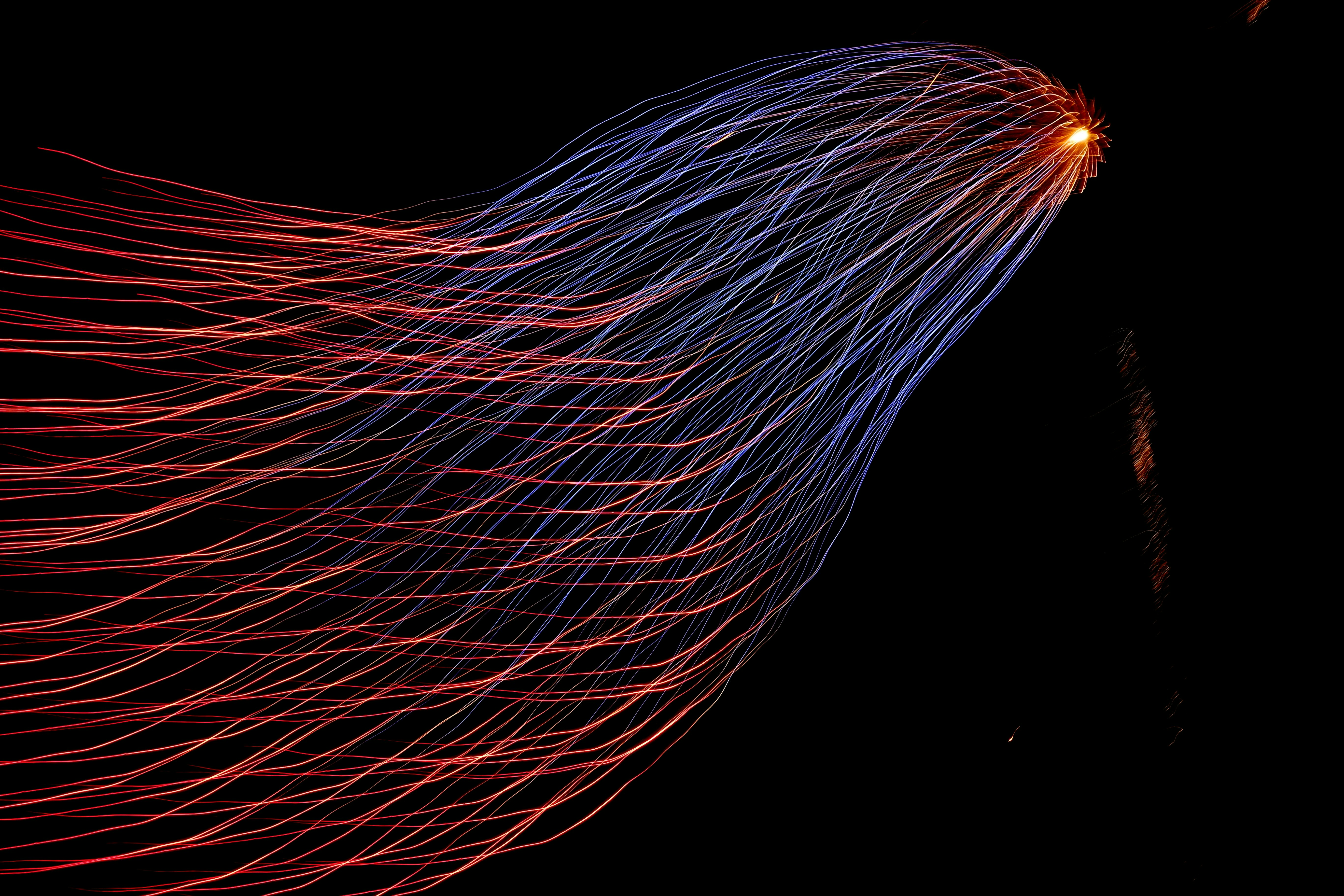# Data Analysis and Visualization with Python

6
4

This course is an introduction to Data Analysis & Visualization using Python. In this course you will get to know different libraries like numpy, pandas, matplotlib, seaborn, plotly, folium etc. and see how to use them to analyze a dataset. At the end of the course you will build an "Exploratory Data Analysis" project on a real-world data.

### Numerical Computing with Numpy

• Arrays, vectors, and matrices in Numpy
• Array operations, slicing, and broadcasting
• Reading from and writing to CSV files

### Exploring Numpy Functions

• Explore the Numpy documentation website
• Demonstrate usage 5 numpy array operations
• Publish a Jupyter notebook with explanations

### Analyzing Tabular Data with Pandas

• Querying, filtering, and sorting data frames
• Grouping and aggregation for data summarization
• Merging and joining data from multiple sources

### Pandas Data Analysis Practice

• Query and sort data from data frames
• Group, merge, and aggregate data frames
• Fix missing and invalid values in data

### Visualization with Matplotlib & Seaborn

• Basic visualizations with Matplotlib
• Tips for customizing and styling charts

### Interactive Visualization with Plotly & Folium

• Creating interactive graphs with Plotly
• Markers, 3D charts, and animation
• Plotting on maps using Folium

### Data Analysis & Visualization Practice

• Querying and filtering pandas data frames
• Static charts with Matplotlib & Seaborn
• Interactive charts with Plotly & Folium

### Exploratory Data Analysis Case Study

• Data preparation and cleaning with Pandas
• Open-ended exploratory analysis & visualization